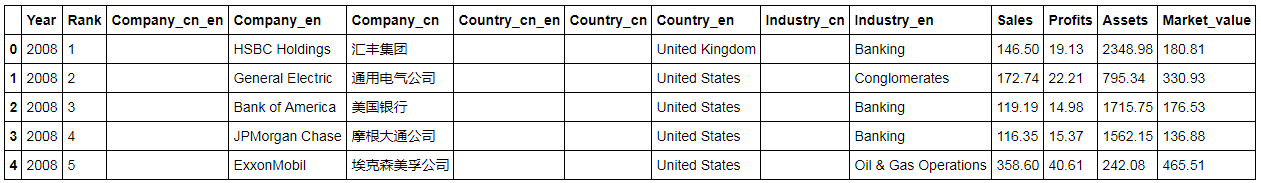# 福布斯系列(6)-数据清洗(b)

projects-forbes Pandas Numpy

## 1 前言

• windows7
• jupyter notebook

## 2 数据清洗的目的

1. 替换NaN值
2. 将字符串型数字转换为数字类型
3. 将数字后面多余的字母等文字剔除
4. 将公司和国家进行拆分
5. 按列名将DataFrame重新排序

the shape of DataFrame:  (2000, 10)

Rank                       int64

Country/area（国家或地区）       object
Industry（行业）              object
Sales （销售额）($bil十亿美元) object Profits （利润）($bil)        object
Assets 资产($bil) object Market Value 市值($bil)    float64
dtype: objectYear               int64
Rank               int64
Company_cn_en     object
Company_en        object
Company_cn        object
Country_cn_en     object
Country_cn        object
Country_en        object
Industry_cn       object
Industry_en       object
Sales            float64
Profits          float64
Assets           float64
Market_value     float64## 3 2008年数据清洗的详细过程

import pandas as pd
import numpy as np



df_2008 = pd.read_csv('./data/data_forbes_2008.csv', encoding='gbk', thousands=',')
print('the shape of DataFrame: ', df_2008.shape)
print(df_2008.dtypes)


df_2008.columns = ['Year', 'Rank', 'Company_en', 'Company_cn','Country_en', 'Industry_en', 'Sales', 'Profits', 'Assets', 'Market_value']


df_2008['Company_cn_en'], df_2008['Country_cn_en'], df_2008['Country_cn'], df_2008['Industry_cn'] = ['','','','']


col_digit = ['Sales', 'Profits', 'Assets', 'Market_value']

for col in col_digit:
# 将数字后面的字母进行替换
df_2008[col] = df_2008[col].replace('([A-Za-z])', '', regex=True)

# 千分位数字的逗号被识别为string了，需要替换
df_2008[col] = df_2008[col].replace(',', '', regex=True)

#将数字型字符串转换为可进行计算的数据类型
df_2008[col] = pd.to_numeric(df_2008[col])


# 按指定list重新将columns进行排序
columns_sort = ['Year', 'Rank', 'Company_cn_en','Company_en',
'Company_cn', 'Country_cn_en', 'Country_cn',
'Country_en', 'Industry_cn', 'Industry_en',
'Sales', 'Profits', 'Assets', 'Market_value']

df_2008 = df_2008.reindex(columns=columns_sort)
print(df_2008.shape)
print(df_2008.dtypes)


## 4 后续

2009年和2010年的数据清洗过程跟2008年基本一致，处理过程就不在本文中描述了（提供源代码供参考）。